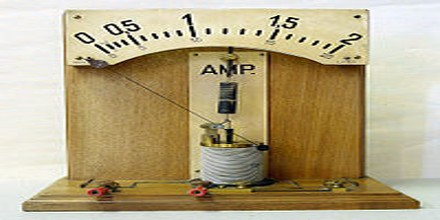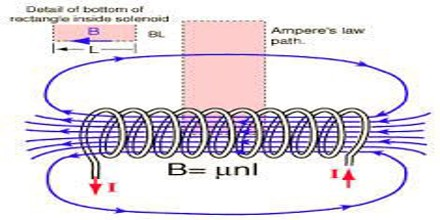About Ampere

Definition

Ampere is the basic unit of electrical current in the International System of Units (SI), equivalent to one coulomb per second. It is also called the unit of electrical current measurement. It is the current that, when passed through a solution of silver nitrate, deposits silver at the rate of 0.001118 gram per second. 1 international ampere equals 0.999835 ampere. SI defines the ampere in terms of other base units by measuring the electromagnetic force between electrical conductors carrying electric current.French mathematician and physicist who is best known for his analysis of the relationship between magnetic force and electric current. He formulated Ampère’s law, which describes the strength of the magnetic field produced by the flow of energy through a conductor. The ampere unit of electric current is named for him.

The ampere is that constant current which, if maintained in two straight parallel conductors of infinite length, of negligible circular cross-section, and placed one metre apart in vacuum, would produce between these conductors a force equal to 2×10−7 newtons per metre of length. The standard ampere is most accurately realized using a watt balance, but is in practice maintained via Ohm’s law from the units of electromotive force and resistance, the volt and the ohm, since the latter two can be tied to physical phenomena that are relatively easy to reproduce, the Josephson junction and the quantum Hall effect, respectively.Ampere’s Law Applcation

Ampère’s force law states that there is an attractive or repulsive force between two parallel wires carrying an electric current. This force is used in the formal definition of the ampere. The electric field due to any known charge distribution ρ(x, y, z) may always be obtained from the Coulomb law it’s a universal tool but the actual calculation is often rather difficult. By comparison, the Gauss law can gives us the electric fields of a sufficiently symmetric charge configuration on a silver platter, without any hard work, but it would not do us much good without symmetry. In magnetostatics, the role of the Coulomb law is played by the Biot–Savart–Laplace law: it works for all steady currents, but the actual calculation can be quite painful.The ampere was originally defined as one tenth of the unit of electric current in the centimetre–gram–second system of units. That unit, now known as the abampere, was defined as the amount of current that generates a force of two dynes per centimetre of length between two wires one centimetre apart. The size of the unit was chosen so that the units derived from it in the MKSA system would be conveniently sized.

Reference: Wikipedia, Dictionary.com, miniphysics.com